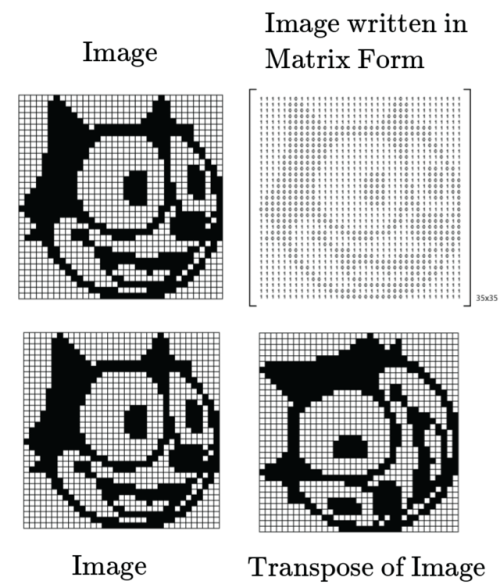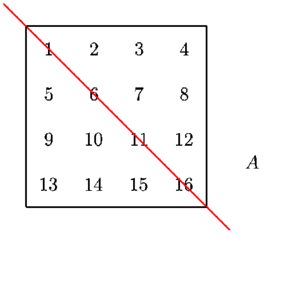## How does one get this idea [for the proof of S…

How does one get this idea [for the proof of Sylvester’s Theorem]? The answer is: I don’t know! It is like asking: How did Michelangelo do this?

## Mathematics is the taming of the infinite

Mathematics is the taming of the infinite

## What is so important about Matrices being Hermitian ?

In linear algebra, an eigenvector  of a linear transformation is a non-zero vector
that only changes by a scalar factor (its eigenvalue) when that linear transformation is
applied to it.

Now for the sake of simplicity lets assume that Energy* as a linear transformation, and when it acts on some position (x1,x2) gives you the energy at that point (e0).

(x1,x2) – Eigenvector, e0 – Eigenvalue.

This e0 that you get is a physical measurable quantity and you do not want this value to be complex. Why ? Complex energies are not a thing of the real world.

And the reason why Hermitian matrices are important in Physics is because if a Matrix is hermitian, then it has real eigenvalues.

* It need not be Energy, it could be any physically measurable quantity. We have just taken energy as an example here.

** A Hermitian matrix (or self-adjoint matrix) is a complex square matrix that is equal to its own conjugate transpose ( A = A )

## Source: Linear Algebra Done RightSource: Linear Algebra Done Right

## fuckyeahphysica: On the transpose of a matrix In this post, I…## On the transpose of a matrix

In this post, I would just like to highlight the fact an image can be represented in a matrix form and matrix transformations such as transpose, shearing, scaling, etc, from an image processing point of view are purely physical !

Check out this article from the klein project if this post interested you.

Have a great day!

And for the sake of completion, I would like to also add this compilation to the post:

## On the transpose of a matrixIn this post, I would just like to…## On the transpose of a matrix

In this post, I would just like to highlight the fact an image can be represented in a matrix form and matrix transformations such as transpose, shearing, scaling, etc, from an image processing point of view are purely physical !

Check out this article from the klein project if this post interested you.

Have a great day!

## Hey there! I was wondering if you knew any good (i.e. solid, complete, thorough) linear algebra book? I’d ask a Math graduate, but I’d rather ask a physicist since that’s what I’m doing myself. PS: are the Russians any good? Bc I tend to favor them when it comes to formal science. For instance, I go to Piskunov when dealing with Calculus.

Hey! I’ve used the Serge Lang book in undegrad school, which has, I think, pretty much everything one needs to get a solid basis in linear algebra. It seems fitting to me for both mathematicians and physicists. I liked it because, while keeping things clear and forward, it has a ton of definitions, plus a lot of interesting appendices. Also it’s not a massive book in terms of volume, which I always appreciate. The thing is, it’s rigorous but kind of basic, you know, the stuff you need to get the state space in quantum mechanics, or matrix calculus, but as far as I remember it lacks a proper introduction to groups and group theory. For that, the Cornwell is the best stuff around if you’re a physicist Have not studied on any Russian authors to be honest, so I wouldn’t know about that, sorry. I only know the Kolmogorov book for Functional Analysis, but nothing on linear algebra.

## Basis Vectors are instructions!

Basis vectors are best thought of in the context of roads.

Imagine you are in a city – X which has only roads that are perpendicular to one another.

You can reach any part of the city but the only constraint is that you
need to move along these perpendicular roads to get there.

Now lets say you go to another city-Y which has a different structure of roads.

In this case as well you can get from one part of the city to any other,
but you have to travel these ‘Sheared cubic’ pathways to get there.

Just like these roads determine how you move about in the city,

Basis
Vectors encode information on how you move about on a plane.

What do I
mean by that ?

The basis vector of City-X is given as:

This to be read as – “ If you would like to move in City-X you
can only do so by taking 1 step in the x-direction or 1 step in the
y-direction ”

The basis vector of City-Y is given as:

This to be read as – “ If you would like to move in City-Y you can
only do so by taking 1 step in the x-direction or  1 step along the
diagonal OB ”

## Conclusion:

By
having the knowledge about the Basis Vectors of any city, you can travel
to any destination by merely scaling these basis vectors.

As an
example, lets say need to get to the point (3,2), then in City-X,  you
would take 2 steps in the x-direction and 3 steps in the y-direction

And similarly in City-Y, you would take 1 step along the x -direction and 2 steps along the diagonal OB.

Destination Arrived 😀